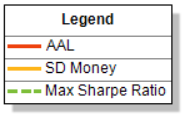# The Optimal RidgeThe 'Optimal Ridge' is a function of the Sharpe ratio and allows the portfolio manager to quickly evaluate optimal portfolio performance for a range of possible portfolio premiums. It is derived from the 'efficient surface' (Please follow the link if you are not already familiar with this concept).

Sharpe Ratio

Although one portfolio can reap higher returns than its peers, it is only a good investment if those higher returns do not come with too much additional risk. Nobel laureate William F. Sharpe devised a ratio to measure portfolio performance. The ex-ante Sharpe ratio formula is as follows (Sharpe Ratio, n.d.):

• Let S be the Sharpe ratio

• Let μ be the expected rate of return of the portfolio

• Let r be the risk free rate

• Let σ be the standard deviation of the rate of return of the portfolio.

Then:

S = (μ - r) / σ

For loss modelling purposes, we have no requirement for the risk-free rate. Hence:

S = μ / σ

In figure 1 below, the blue marker on the efficient branch of the efficient frontier shows the location of the maximum Sharpe ratio (Smax). When considering which efficient portfolio to choose from the efficient frontier, the portfolio with the maximum Sharpe ratio is a good place to start.

Figure 1: The Efficient SurfaceAs long as the chosen efficient portfolio is located on the efficient branch of the efficient frontier – no choice of portfolio is incorrect. It is very much a question of risk appetite. However, Smax represents the optimal blend of risk vs return.

Mount Sharpe

Mount Sharpe (figure 2) derives from the efficient surface and is a plot of (μ, S, P) where S = μ / σ

Figure 2: Mount Sharpe• The greater a portfolio's Sharpe ratio, the better it's risk to return ratio.

• There exists an optimal ridge. That is, for each premium amount there exists a maximum Sharpe ratio corresponding to a particular 𝜇.

• There exists a peak Sharpe ratio at a specific (𝜇, P) co-ordinate. Hence the surface has been labelled 'Mount Sharpe.'

The Optimal Ridge

For each premium amount, there exists a maximum Sharpe ratio corresponding to a particular 𝜇. Figure 2 clearly shows the profile of Mt. Sharpe as P increases from Pmin to Pmax. The side profile shows the optimal ridge andthis is plotted in figure 3 along with the associated portfolio mean loss (AAL) and standard deviation of the loss in monetary amounts.

Figure 3: The Optimal RidgeThe resultant 2D chart is easier to visualise and interpet and gives a quick snapshot of the 'best' portfolio metrics for the range of possible portfolio premiums. Notice how the portfolio performance (as measured by the max sharpe ratio) drops off as more premium is required from the portfolio. This is because as more premium is demanded from the portfolio, more contribution must be made from the more volatile/correlated/lower return contracts (that can be exluded/minimised for lower premiums).

The associated portfolio statistics are displayed in table 2. (Table 1 shows the current portfolio statistics by way of comparison).

Table 1: Pre-optimised portfolio statisticsTable 2: The Optimal Ridge (Max Sharpe) Table of ValuesRiskWave solves the efficient surface quickly and then computes the optimal ridge instantaneously. This allows the portfolio manager to quickly evaluate portfolio performance according to the total written portfolio premium. The portfolio manager can be assured that the ratio of risk to return is optimal for the given portfolio premium amount. The contract percent shares which comprise any given portfolio can be extracted at the click of a button.

Similar 'ridges' can be generated for the minimum risk and maximum return case. Further, a maximum 'return on capital' optimal ridge can be generated from the associated VaR surface. This is useful when the PM is particularly interested in key return period losses.

See All